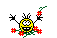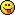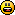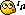Author Topic: Gamepad Test  (Read 4891 times)matchy

• Prof. Inline
••• Posts: 1545« on: 2014-Aug-10 »
So I finally got a USB PC/Linux gamepad for the first time, a Logitech F310.It's cool and works well, so I had to write a test tool and included some compensation trimming as I noticed that the left and right, x & y axis of the analog sticks don't spring back to center sometimes (eg. 0.05 at worst).I'm not sure if this is hardware or software as the springy z triggers always return to zero.I was hoping to use it on Adnroid but when I test it only returns the accelerometers.

Code: (GLBasic) [Select]
// --------------------------------- //
// Start: Sunday, August 10, 2014
// IDE Version: 12.096

TYPE _font
num
width
height
ENDTYPE

TYPE _sprite
num
width
height
ENDTYPE

TYPE _screen
width
height
width2
height2
backcolor
font AS _font
sprite_id

FUNCTION init:
SETSCREEN 800, 600, 0
GETSCREENSIZE self.width, self.height
self.width2 = self.width / 2
self.height2 = self.height / 2
self.backcolor = 0x000000
self.font.num = GENFONT()
LOCAL file\$ = "Media/font.png" // make a font file!
IF DOESFILEEXIST(file\$)
SETFONT self.font.num
ENDIF
GETFONTSIZE self.font.width, self.font.height
ENDFUNCTION
ENDTYPE

TYPE _joy
a = 0
b = 1
x = 2
y = 3
lb = 4
rb = 5
back = 6
start = 7
l = 8
r = 9

jx = 0
jy = 1
jz = 2
rx = 3
ry = 4
rz = 5
dx = 6
dy = 7

count
stick[] AS _stick

FUNCTION init:
self.count = GETNUMJOYSTICKS() - 1
IF self.count > -1
DIM self.stick[self.count + 1]
FOR i = 0 TO self.count
self.stick[i].id = i
DIM self.stick[i].button
DIM self.stick[i].control
FOR j = 0 TO 31
SELECT j
CASE joy.a
self.stick[i].button[j].name\$ = "A (Green)"
CASE joy.b
self.stick[i].button[j].name\$ = "B (Red)"
CASE joy.x
self.stick[i].button[j].name\$ = "X (Blue)"
CASE joy.y
self.stick[i].button[j].name\$ = "Y (Yellow)"
CASE joy.lb
self.stick[i].button[j].name\$ = "Left Top"
CASE joy.rb
self.stick[i].button[j].name\$ = "Right Top"
CASE joy.back
self.stick[i].button[j].name\$ = "Back"
CASE joy.start
self.stick[i].button[j].name\$ = "Start"
CASE joy.l
self.stick[i].button[j].name\$ = "Left Joy"
CASE joy.r
self.stick[i].button[j].name\$ = "Right Joy"
DEFAULT
self.stick[i].button[j].name\$ = "?"
ENDSELECT
NEXT
FOR j = 0 TO 7
SELECT j
CASE joy.jx
self.stick[i].control[j].name\$ = "Left Joy X"
CASE joy.jy
self.stick[i].control[j].name\$ = "Left Joy Y"
CASE joy.jz
self.stick[i].control[j].name\$ = "Left Joy Z"
CASE joy.rx
self.stick[i].control[j].name\$ = "Right Joy X"
CASE joy.ry
self.stick[i].control[j].name\$ = "Right Joy Y"
CASE joy.rz
self.stick[i].control[j].name\$ = "Right Joy Z"
CASE joy.dx
CASE joy.dy
DEFAULT
self.stick[i].control[j].name\$ = "?"
ENDSELECT
NEXT
NEXT
ENDIF
ENDFUNCTION

FUNCTION show:
WHILE TRUE
IF self.count > -1
// FOREACH js IN joy.stick[]
self.stick.update()
self.stick.view()
// NEXT
ELSE
PRINT "No joystick found. Connect one and restart!", 0, 0
self.init()
ENDIF
SHOWSCREEN
WEND
ENDFUNCTION
ENDTYPE

TYPE _button
value
name\$
press
last_value
ENDTYPE

TYPE _stick
id
trm = 0.055
control[] AS _button
button[] AS _button

FUNCTION trim:
LOCAL val
FOR i = 0 TO 5
val = self.control[i].value
IF (val < 0 AND val > -self.trm) OR (val > 0 AND val < self.trm)
val = 0.0
ENDIF
IF val = 0.0
self.control[i].value = 0.0
self.control[i].press = 0
ELSE
INC self.control[i].press
ENDIF
self.control[i].value = val
NEXT
ENDFUNCTION

FUNCTION update:
self.control[joy.jx].value = GETJOYX(self.id)
self.control[joy.jy].value = GETJOYY(self.id)
self.control[joy.jz].value = GETJOYZ(self.id)
self.control[joy.rx].value = GETJOYRX(self.id)
self.control[joy.ry].value = GETJOYRY(self.id)
self.control[joy.rz].value = GETJOYRZ(self.id)
self.control[joy.dx].value = GETDIGIX(self.id)
self.control[joy.dy].value = GETDIGIY(self.id)
FOR i = 0 TO 31
self.button[i].value = GETJOYBUTTON(self.id, i)
IF self.button[i].value <> 0
INC self.button[i].press
ELSE
self.button[i].press = 0
ENDIF
NEXT
trim()
ENDFUNCTION

FUNCTION view:
LOCAL x = 0, h = screen.font.height, y = 0, c
FOR i = 0 TO 7
IF self.control[i].value <> 0
c = 0x00ff00
ELSE
c = 0x000022
ENDIF
x = screen.width * 0.05
DRAWRECT x - h, (i * h), h - 1, h - 1, c
PRINT self.control[i].name\$ + ":", x, i * h
x = screen.width * 0.3
PRINT FORMAT\$(3, 3, self.control[i].value), x, i * h
x = screen.width * 0.435
PRINT self.control[i].press, x, i * h
NEXT
FOR i = 0 TO 15 //31
IF self.button[i].value <> 0
c = 0x00ff00
ELSE
c = 0x000022
ENDIF
x = screen.width * 0.55
DRAWRECT x - h, (i * h), h - 1, h - 1, c
PRINT self.button[i].name\$ + ":", x, i * h
x = screen.width * 0.8
PRINT self.button[i].value,   x, i * h
x = screen.width * 0.88
PRINT self.button[i].press,   x, i * h
NEXT
plot()
ENDFUNCTION

FUNCTION plot:
LOCAL jx = self.control[joy.jx].value
LOCAL jy = self.control[joy.jy].value
LOCAL jz = self.control[joy.jz].value
LOCAL rx = self.control[joy.rx].value
LOCAL ry = self.control[joy.ry].value
LOCAL rz = self.control[joy.rz].value
LOCAL s = (10 * jz) + 20, t = (s / 2)
DRAWRECT (jx * screen.width2) + screen.width2 - t, (jy * screen.height2) + screen.height2 - t, s, s, 0x00ffff
DRAWRECT (rx * screen.width2) + screen.width2 - t, (ry * screen.height2) + screen.height2 - t, s, s, 0xffff00
ENDFUNCTION
ENDTYPE

GLOBAL screen AS _screen
GLOBAL joy AS _joy
screen.init()
joy.init()
joy.show()
« Last Edit: 2014-Aug-10 by matchy »erico

• Community Developer
• Prof. Inline
••• Posts: 4397« Reply #1 on: 2014-Aug-10 »
Good, I have a logitech dualshock, it is pretty similar and I tell you this things last forever. Really well built.

About the test, I get different names for the buttons and controls.
Very rarely the left analog x axis won´t return to zero from -1, the other direction is fine.
But it is probably because my joy is ancient.

Like I stated on another thread, if I change joy mode, like switch analog/pad, then the pads won´t give a 1 ,-1 value but almost that.
Mr.Tatoad said it is normal for hardware to respond this way.

I have to try this code on caanoo sometime.matchy

• Prof. Inline
••• Posts: 1545« Reply #2 on: 2014-Aug-10 »
Oh no ... I didn't calibrate it so I'll try that next time.Does your gamepad have a X mode / DirectX mode switch underneath? This swaps a few buttons around but I haven't tested in D mode fully yet. There also a mode button on top that switches the d-pad and the left analog stick for 0 / -1 or ratio.erico

• Community Developer
• Prof. Inline
••• Posts: 4397« Reply #3 on: 2014-Aug-10 »
Mine only has the mode button on top of it (the dpad/analog switch). And when it is on (it has its own led) the pad reports on the analog as expected but mine goes -0.992 to 0.992 only.

I said on the post before that my analog rarely gets stuck one direction, well, I have been trying it out for a while and it dosen´t happen anymore, so I guess it was because I didn´t use it for a while and some dirt got in the way.

Still, it is always good to add some small threshold.

As for android, the joy command was supposed to bring the accelerometer values, but last time I tried there was a bug, I´m not sure if it is fixed on latest version.
But on an old version, it did work really fine. Way more sensible then the likes of accelerometer on the caanoo.
Also, you could probably read the joypad with Android Extras if you can connect it to an android device.spacefractal

• Community Developer
• Prof. Inline
•• Posts: 3958